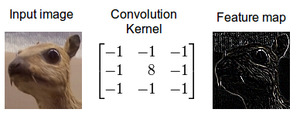•## Understanding Convolution in Deep Learning

Convolution is probably the most important concept in deep learning right now. It was convolution and convolutional nets that catapulted deep learning to the forefront of almost any machine learning task there is. But what makes convolution so powerful? How does it work? In this blog post I will explain convolution ...

• ## Convolution Is Fancy Multiplication

Like making engineering students squirm? Have them explain convolution and (if you're barbarous) the convolution theorem....

•## A technical report on convolution arithmetic in the context of deep learning

A technical report on convolution arithmetic in the context of deep learning